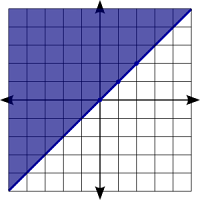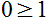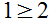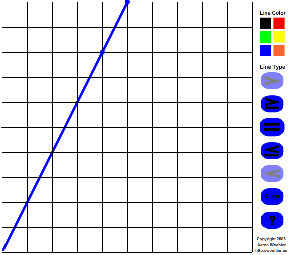## Sunday, December 30, 2007

### Math Facts Question Generator

Here it is I finally finished the the Math Facts Question Worksheet generator. With a few adjustments I was able to adapt most of the features of the Moodle Math Question generator to be able to create a printable version with an answer key. This program will create a set of math fact questions based on the users input parameters. At this point this version is limited to addition, subtraction, multiplication, and division.

Highlights:

• Vertical or horizontal problem formatting.
• Each time that the page is requested the order of the questions is changed.
• The answer key prints on a separate page.
• Customizable directions
• Custom key/worksheet identifier.
• Integer only answers for division problems.
• Positive answers for subtraction problems.
Check it out

## Tuesday, December 18, 2007

### Graphing website

While tutoring an Algebra 2 student I stumbled upon this website that looks pretty useful and seems to work pretty well. Thanks Zona.

EZ Graph @ http://id.mind.net/~zona/ezGraph/ezGraph.html

## Friday, December 14, 2007

### Graphing Systems of Equations

The goal of solving systems of equations is to determine if two or more lines intersect and if they do where do they intersect. This lesson will only deal with two lines.

First we need to determine how the lines of the two equations relate to each other. Do they intersect, are they parallel, or are they the same line. The mathematical ways of describing this is are they consistent, inconsistent, dependent, and independent. There are three options as shown in the picture below: To find the answer to the system you need to ask one or two questions depending on the problem.

Parallel lines: Independent and inconsistent

Question 1: First write the equation in slope intercept form. Then ask the question is the slope in each equation the same or different? If it is the same then they are parallel and you need to proceed to the next question. If it is different then the point at which the lines cross is the solution to the system and we are done.

Question 2: Since the slope is the same the lines are parallel. Now you need to ask the question "Are they the same line?" If the y-intercepts are the same then they are the same line and there is an infinite number of solutions. The line is the solution to the system of equations because it represents all of the points that work in both equations which are really the same equation just written differently.

If they are not the same then there is no solution, that is the lines do not cross.

Practice Problems:

Find the solution of the equations y = 2x + 4 and y = 0.5x - 2

First we recognize that the equations are in slope intercept form (y = mx + b) and that the slopes of each of the lines are different so we know that they will cross at some point. The next step is to graph the line. It is very important that your lines be accurate so I would recommend placing as many calculated points as possible on the graph for each line.

The solution is ( -4, -4 ). Make sure to check your answer by plugging the point into both problems.
y = 2x + 4
-4 = 2 ( -4) + 4
-4 = -8 + 4
-4 = -4

and

y = 0.5x - 2
-4 = 0.5 (-4) - 2
-4 = -2 - 2
-4 = 4

Lets try another example:
Solve the system of equations:
y= 4
2x - 3y = -6

First off notice that the second equation is in standard form and not slope intercept form. We need to rearrange it so that we can compare the slopes.
2x - 3y = -6
Subract 2x from both sides.
-3y = -2x - 6
Now divide both sides by -3
y = (2/3)x + 2

Again notice that the slopes are not the same so we know that they will cross at some point. Graph your line, making sure to plot lots of points to keep it accurate.The solution is ( 3, 4). Check your answer
y = 4
4 = 4. Remember that this equation doesn't care about the x value so it can be anything.

2x - 3y = -6. Always use the original problem in case you made a mistake when you were changing the problem to slope-intercept form.
2(3) - 3(4) = -6
6 - 12 = -6
-6 = -6

## Monday, December 10, 2007

### How to Graphing Inequalities in the Coordinate Plane.

Objective:
• Graph inequalities in a xy coordinate graph.

Assumptions:
• Ability to graph a line using the slope-intercept form (y = mx + b)

Concepts:

• The shaded area of a graph represents all of the coordinates that will work in a given equation.
• A solid edge of the shaded area means that the edge is part of the solutions to the equation.
• A dashed edge of the shaded area means that the edge of the graph is not part of the solutions.

Directions:
Graph the equationStep 1: Draw the graph just as you would y = x . This equations in slope intercept form would look like this. The 0 means that you will go through the origin, place a point there. Now use the slope to draw the rest of the line. From the origin go up one and to the right one and place another point. Repeat until you have several points.Now draw a solid line because the equation to be graphed is greater than or equal to. Your graph should now look like this:Step 2: Next shade everywhere above the line because the equation states that the y values are greater than or equal to the line for any given x value.Does the point ( 1, 2) work in the equation? yesDoes the point ( -1, 0) work in the equation? yesDoes the point ( 1, 0) work in the equation? noDoes the point ( 2, 1) work in the equation? noLets try another one.

Graph graph y > 2x + 3
Remember the steps: plot some points, draw the line (solid if equal to, dashed if greater than or less than), shade above with greater than, shade below with less than.
The line will cross the y axis as 3 then go up 2 and over 1 for the slope. Start by placing a point at 3 on the y axis. Next use the slope to place 2 more dots, then make a dashed line through the dots.
The equation uses the greater than inequality so it should be shaded above the line.

Now that we have the common ones out of the way lets look at the ones that may trip you up such as the ones with only one variable like y > 2 and x < -3.

Graph y > 2
Remember that is just a horizontal line. This is just a horizontal line that is shaded above the line and dashed because it is not equal to the line it is only greater than the line.

Graph x < -3

Remember that is just a vertical line. This is just a vertical line that is shaded to the left of the line and dashed because it is not equal to the line it is only less than the line. The x values on the left are less than the line.

Things to remember when graphing inequalities:
Solid line and shaded above the line.
Solid line and shaded below the line
> Dashed line and shaded above the line

y > # Horizontal line and shaded above the line
y < # Horizontal line and shaded below the line
x > # Vertical line and shaded on the right side of the line
x < # Vertical line and shaded on the left side of the line.

## Tuesday, December 4, 2007

### Random Student Selector

I my Algebra I class the other day I was using the Quizdom pick option to select my students to answer questions, during the class period 2 of my 11 students were selected 3 times while 2 others were not selected at all. Out of about 10 questions this wasn't very even percentages. One of the students Nathan J., who is also one of my computer programming students, and I were discussion this. We came up with a way to decrease the likelihood that someone would be selected once they have been selected. We chose this because if you eliminate the student until all have been selected the student will then mentally check out. With our solution the student can still be selected but the likelihood of them being selected twice in a row is reduced. Given enough time the Quizdom solution would be fine but if you are only asking 10 questions it is important that the student selection should be random and balanced. You can check out our free implementation of the student selector at http://www.aschool.us/moodle-scripts/

With this online implementation you simply enter a list of names, one per line. Go to the next page and start selecting students. Simply refreshing the page will select a new students name. The names are written in a large font so that it can be used in class.

An option that I have thought of adding would be to create a random list of the names so that it could printed to be used away from the computer and would still have the randomness for the students. If you are interested in this option please leave a comment. Since I have access to the Internet in my classroom I don't see this as a necessary feature for myself but if someone else would use it I could easily add it.

## Thursday, October 25, 2007

### General usage flash grapherThe need arose to be able to quickly graph points and lines during class presentations so I decided to develop the following flash application. The curve is a great addition but I don't know that it will be as useful. It's pretty simple to use just select the point, line, or curved button. Next put them on the graph. If you mess up just hit the red X and it all goes away. Below is a screen shot of the program. Just give it a click to head on over to my other website where you can give it a try. Feel free to come back and leave me some comments on how useful you thing this is.

## Wednesday, October 24, 2007

### Learning Math & Science Applets

Check out this great website that can help your students understand different math and science topics. The site is not free but they do have a 30 trial and you can try out the applets for 5 mintues before they are disabled.
I introduced it to my students today I'll provide some updates here on whether they find it useful or not.

explorelearning.com

## Tuesday, October 23, 2007

### Real world application

It appears that the people at NASA have done a great job at making interactive learning pages for a variety of topics. Check them out at spaceplace.nasa.gov/en/kids/
Some of the topics include weather, lasers, CCD's (camera's), robitics, coordinate systems, and more.

### Great Chemistry Sites

While searching the Internet yesterday I realized the abundance of interesting interactive periodic chart websites. Here are a couple of them that I thought looked pretty useful and well designed.
I'm sure that there are many more great sites out there theres are just a couple that caught my interest. Make sure and look at Quizlet.com it appears to be a great teaching to for other classes as well.

### New School Bells Program

My school has been using an old version of windows that used a NI input/output board. The computer finally crashed and I wasn't able to get the driver updated so I decided to develop new software that did not depend on the NI board. Having done some research on this before I decided to develop a solution that would use the parallel port because it would be easy to program. The design needed to be such that it could be easily ported to a standard serial port or USB port. Talking with the registrar at school we agreed on the following list of features as important.
• Easy to use
• Dependable
• Manual over ride
• Synchronize time with an Atomic clock or Internet
• Select which days to ring on, no weekends, etc....
• Save predefined schedules
Putting the pieces together
• Linux is the new OS of choice for dependability and the ability to remotely access the system. Also the program will try to access a database to get the times for the bell that day. If it fails it will try to access a file on the local computer. Access can be given to these files through file sharing should the database fail. Also Linux can be run on most any old computer.
• The decision was made to integrate the UI with the schools web based attendance system. This would take care of access for multiple users and security. This would also take care of the ease of use because the registrar is already used to the system.
• The registrar or whoever is given permission to change the bells can create and update schedules and then assign the schedules via a monthly calendar to any day of the year.
• The hardware for the moment is a parallel port connected to a relay. There is a manual button that can ring the bells and an override to keep the computer from ringing the bells. This is handy because the bells ring while the computer is booting. A future solution will be a device that works via the serial or USB port. I have a friend that has developed a independent bells system that can be programmed via the serial or USB port which we hope to integrate into our system because it will make it that much dependable since it could continue to run independent of the computer.
This program is still under development and is being used by my school. If you are interested in this program post a comment and I'll try and post a copy of the program when it becomes available.

## Friday, June 1, 2007

### Moodle Math Question Generator

I've created a numeric question generator for Moodle that will create MoodleXML files that can be imported into your course.
It will create addition, subtraction, multiplication, division, powers, and square root problems based on the upper and lower bounds of numbers that you give it. It can also limit the answers to only positive numbers and/or only integer answers.
Check it out here: http://aschool.us/moodle-scripts/math-questions.php

## Thursday, March 8, 2007

### Finding the Greatest Common Factor

Here is a little flash file that I created this morning to help you understand how to find the greatest common factor between two numbers. I hope to add audio to this later but don't have the time at the moment. Simply click the button at the bottom to work your way through the process.
To find the greatest common factor between 18 and 24 first you need to find the prime factorization of each. To do this divide 18 by 2 and you get 9. Nine is not a prime, it can be divided by 3. So the prime factorization of 18 is 2x3x3. Next do the same with 24. Twenty-four divided by 4 is 6. Neither 4 or 6 are prime so they need to be broken down farther. Four is divisible by 2 and 6 is divisible by 2 and 3. Making the prime factorization of 24 = 2x2x2x3. Next find the common numbers that are in each prime factorization of each number. Both 18 and 24 contain a 2 and a 3. Multiply these together to get 6. Thus 6 is the greatest common factor of 18 and 24. To download download the file flash file can be downloaded from to www.woehler.us

## Friday, January 26, 2007

### New moodle flash question type

I've finally release the first version of my new question type that uses the Student Line Grapher to implement a new Moodle question type that I made to record the answers of students responses to equations and inequality graphs.

My goal was to make a question type that could be adapted to different types of questions depending on the flash module that was used. The possibilities are endless: line graphs, plotting points, graphing shapes and there transformations, polynomial graphs, etc.... The beauty of my design is that it can be easily changed by simply changing the flash module as long as you can make the flash module come up with a text answer.

This version is a first rough draft so I'm hoping to get some good feedback and be able to make lots of improvements.

http://moodle.org/mod/forum/discuss.php?d=63471

## Wednesday, January 17, 2007

### Graphing Resources

I've created a set of resouces that can help you create graphing materials. The were created using Inkscape a open-source vector drawing program. The files are saved as svg, pdf, gif, and png formats. You will find a ready made set of graph paper and individual graphs that can be inserted into word, open office, a web page or where ever you want. I've also provided the original svg files so that you can quickly make any changes that you want.

You can find them on my website at www.woehler.us. Let me know if you find these to be useful.

## Tuesday, January 16, 2007

### Student Line Grapher

While working with my Algebra 1 students to try and teach them how to graph lines. I came up with the idea to create a flash module that would work with moodle to record student's graphs. This idea came out of my previous project which was to create a quick way to draw graphs while lecturing.

This module is pretty slick you can use it to teach students how to graph linear inequalities and equality graphs. My other web page explains the details but here are the basics.

Select your line type, which is by default solid for equality next click to points that are on the line. The flash module extends the line to the edge of the graph. If you are going to graph an inequality then click on shading. Click to points on the line and then which side of the line should be shaded.

You will notice that when you select shading you now have the option of two different line types. The dashed line is for inequality graphs.

Here is the really cool part though. When you create the graph, if you set a value in the flashvars variable the equation of the line can be recorded to a form value in the web page which can then be submitted for grading.

I've implemented this version with moodle but I'll post that information later since I'm not using the latest version of moodle and want to cleaned up the code a little bit and make sure that it is compatible with the latest version.

## Monday, January 8, 2007

### Finished

Well I finally finished my graphing utility. I'm sure there are many cool features that I could add but I don't have time for that at the moment. Please let me know what you think. I've posted a copy at www2.woehler.us/cms/. You can find the flash/exe/hqx version and instructions there.

I hope that this will be a helpful resource to math teachers presenting graphing concepts such as slope intercept form, y-intercept form, standard form, and point slope form or whatever other type of graph you are planning on using. It even works with inequalities.

## Wednesday, January 3, 2007

### Teaching graphingMy algebra students are stuggling with the concept of graphing so I've created a couple of Flash modules that facilitate teaching graphs. One of them you can simply put points on the graph by clicking and it will tell you the coordinates on the graph. The other one allows you to click two points on the graph to draw a line segment and change the line segment into an equality or inequality graph.

This post is just to see if there is some interest in these modules. I'm looking for some math teachers out there that might be interesting in testing it out for me and giving me some feedback before I post it for free on the internet.

You can put this module into a powerpoint, show it in a web page, or run it as a program.